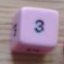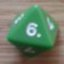Calculate probabilities for a weird dice mechanic?

## Calculate probabilities for a weird dice mechanic?

 Thu Nov 2 01:22:09 2017   by   gaptooth I wonder if I could come up with a way to calculate the probabilities for a dice mechanic for Twisted Tunnels RPG (a Tunnels & Trolls-inspired game).In Twisted Tunnels, the players roll dice pools like in T&T combat. But instead of totaling the numbers on every die, there score is either the single highest number showing OR the sum of any dice showing the same number, whichever is higher.And if they're rolling 2 or more dice and they all match, the player adds them up and rolls again, adding their subsequent score to the previous total. They can keep adding and rolling again as long as the dice all match.Our playtesting has been fun, but I wanted to get a programatic look at the probabilities for different scores given different numbers of dice.Can anyone suggest how to enter such a quirky mechanic into Troll's dice expression language? Is it possible?Thanks in advance! Thu Nov 2 10:28:56 2017   by   Torben Saying that the score is either the single highest number of the largest sum of identical numbers, whichever is higher, is just the same as saying that the score is the highest sum of dice showing the same number -- whether there is only one or several of these.That part alone is easy enough to do in Troll:`x := 3d6;max foreach i in 1..6 do sum (i = x)`where you change 3 to whatever your pool size is.If you want to reroll and add whenever all the dice are the same, you modify the above to this:`function twisted(n) =  x := n d6;  y := max foreach i in 1..6 do sum (i = x);  if (count different x) > 1 then y else y + call twisted(n)call twisted(3)`Note that this version assumes that the pool size is greater than one, as the count of different dice will always be 1 if the pool size is 1. Thu Nov 2 14:46:45 2017   by   gaptooth Thank you! Thu Nov 2 22:48:12 2017   by   gaptooth Thank you! Thu Nov 2 22:48:18 2017   by   gaptooth Thank you!